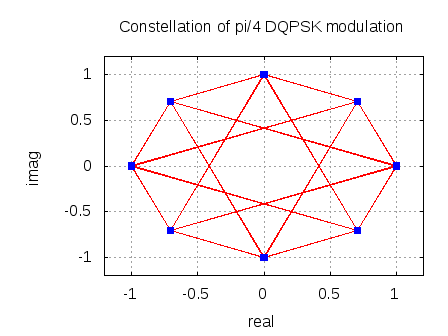- DSP log - http://www.dsplog.com -

Non coherent demodulation of pi/4 DQPSK (TETRA)

Posted By Krishna Sankar On April 12, 2010 @ 8:58 pm In Modulation | 9 Comments

In TETRA specifications , one of the modulation technique used is Differential Quaternary Phase Shift Keying (DQPSK). We will discuss the bit error rate with non-coherent demodulation of DQPSK in Additive White Gaussian Noise (AWGN) channel.

## pi/4 DQPSK

The incoming bit sequence is grouped into two bits and is mapped into differential phase as follows:

 B(2k-1) B(2k) 1 1 0 1 0 0 1 0

Table : Phase transitions for DQPSK modulation (Ref Table 5.1 of ETSI 301-893 V3.2.1 )

The modulation symbol is formed by applying a phase offset to previous symbol and is defined as follows:

and

.

Alternately, the phase transitions can be represented as

.

The constellation of the DQPSK occupies phase values separated by as shown below in the blue dots. The red lines shows all possible phase transitions.Figure: Constellation of DQPSK (Ref Figure 5.1 of ETSI 301-893 V3.2.1 )

## Channel Model

The transmitted waveform gets corrupted by noise , typically referred to as Additive White Gaussian Noise (AWGN).

White : The spectrum of the noise if flat for all frequencies.

Gaussian : The values of the noise follows the Gaussian probability distribution function,

with mean and

variance .

A non-coherent receiver relies on the phase transitions between consecutive symbols to form an estimate of the transmitted bits. The sequence of operation is as follows:

a) On the received symbols estimate the phase

b) Find the delta of the estimated phase between consecutive symbols

c) Quantize the estimated delta phase values lying from as follows and convert to bits per the following encoding:

.

Figure: Received delta phase to bit mapping

The theoretical bit error rate defined with the mapping above is (Ref Chapter 5.2.8 of Digital Communications by Proakis, 4th Edition )
,

where

,

,

and

is the modified Bessel function of first kind.

## Simulation results

The script performs the following

(a) Generate random binary sequence of +1′s and 0′s.

(b) Group two bits together and apply DQPSK modulation

(d) At the receiver, estimate the phase of the received symbols. Based on the delta of the received phase, estimate the transmitted bits

(e) Repeat for multiple values of and plot the simulation and theoretical results.

Figure: BER plot for DQPSK with non-coherent demodulation

## Observations

Can see good agreement between simulated and theoretical results.## Reference

URL to article: http://www.dsplog.com/2010/04/12/non-coherent-demodulation-of-pi4-dqpsk-tetra/

URLs in this post:

 TETRA specifications: http://webapp.etsi.org/WorkProgram/Report_WorkItem.asp?WKI_ID=22928&curItemNr=116&totalNrItems=483&optDisplay=100000&qSORT=HIGHVERSION&qETSI_ALL=&SearchPage=TRUE&qTB_ID=218%3BTETRA&qINCLUDE_SUB_TB=True&qINCLUDE_MOVED_ON=&qSTOP_FLG=&qKEYWORD_BOOLEAN=&qSTOPPING_OUTDATED=&butSimple=Search&includeNonActiveTB=FALSE&includeSubProjectCode=&qREPORT_TYPE=SUMMARY